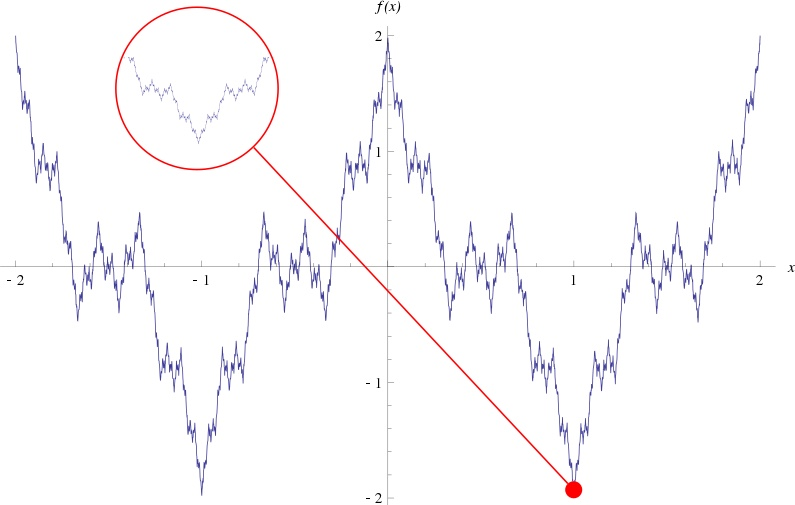## 连续

$\displaystyle\lim_{x\to x_0}{f(x)}=f(x_0)$

## 导数

### 可导

（1）设 f(x)在 $x_0$ 及其附近有定义,则当 $\displaystyle\lim_{\Delta{x}\to0}{\frac{f(x_0+\Delta{x})-f(x_0)}{a}}$ 存在, 则称 f(x) 在 $x_0$ 处可导。
（2）若对于区间 (a,b) 上任意一点 (x，f(x)) 均可导，则称 f(x) 在 (a，b) 上可导。

## 微分

### 定义

$\Delta{y}=f(x+\Delta{x})-f(x)=f’(x)\Delta{x}+\alpha(\Delta{x})\Delta{x}$

### 可微## 连续，可导，可微的关系

• 一元函数：可导必然连续，连续推不出可导，可导与可微等价。
• 多元函数：可偏导与连续之间没有联系，也就是说可偏导推不出连续，连续推不出可偏导。
• 多元函数中可微必可偏导，可微必连续，可偏导推不出可微，但若一阶偏导具有连续性则可推出可微。

## 梯度

f(x+Δx,y+Δy)≈f(x,y)+∂f∂xΔx+∂f∂yΔy

# Referen

http://jermmy.xyz/2017/07/27/2017-7-27-understand-lagrange-multiplier/